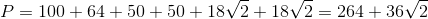# PSAT Math : How to find the perimeter of a polygon

## Example Questions

### Example Question #24 : Other Polygons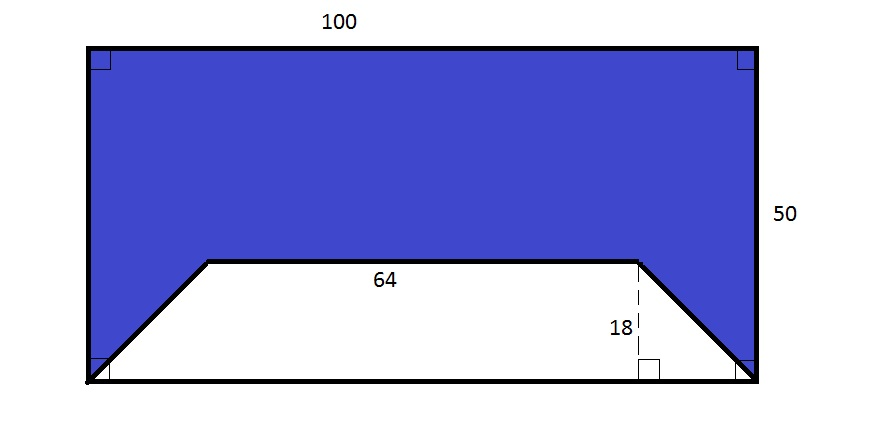Note: Figure NOT drawn to scale.

Refer to the above figure. The white trapezoid is isosceles. Give the perimeter of the blue polygon.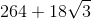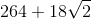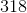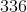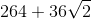The lower base of this trapezoid is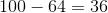units longer than the upper base, and being isosceles, it is symmetrical. As a result, the lower leg of the right triangle at bottom is half this difference, or 18, which is the same as the upper leg. That makes the right triangle isosceles, and, therefore, a 45-45-90 triangle. Subsequently, the hypotenuse, which is one leg of the trapezoid, has lengthtimes a leg of the triangle, or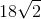The blue polygon has two sides that are the legs of this isosceles trapezoid, both of which have length; its other three sides are of length 64, 50, 100, and 50. Add them: